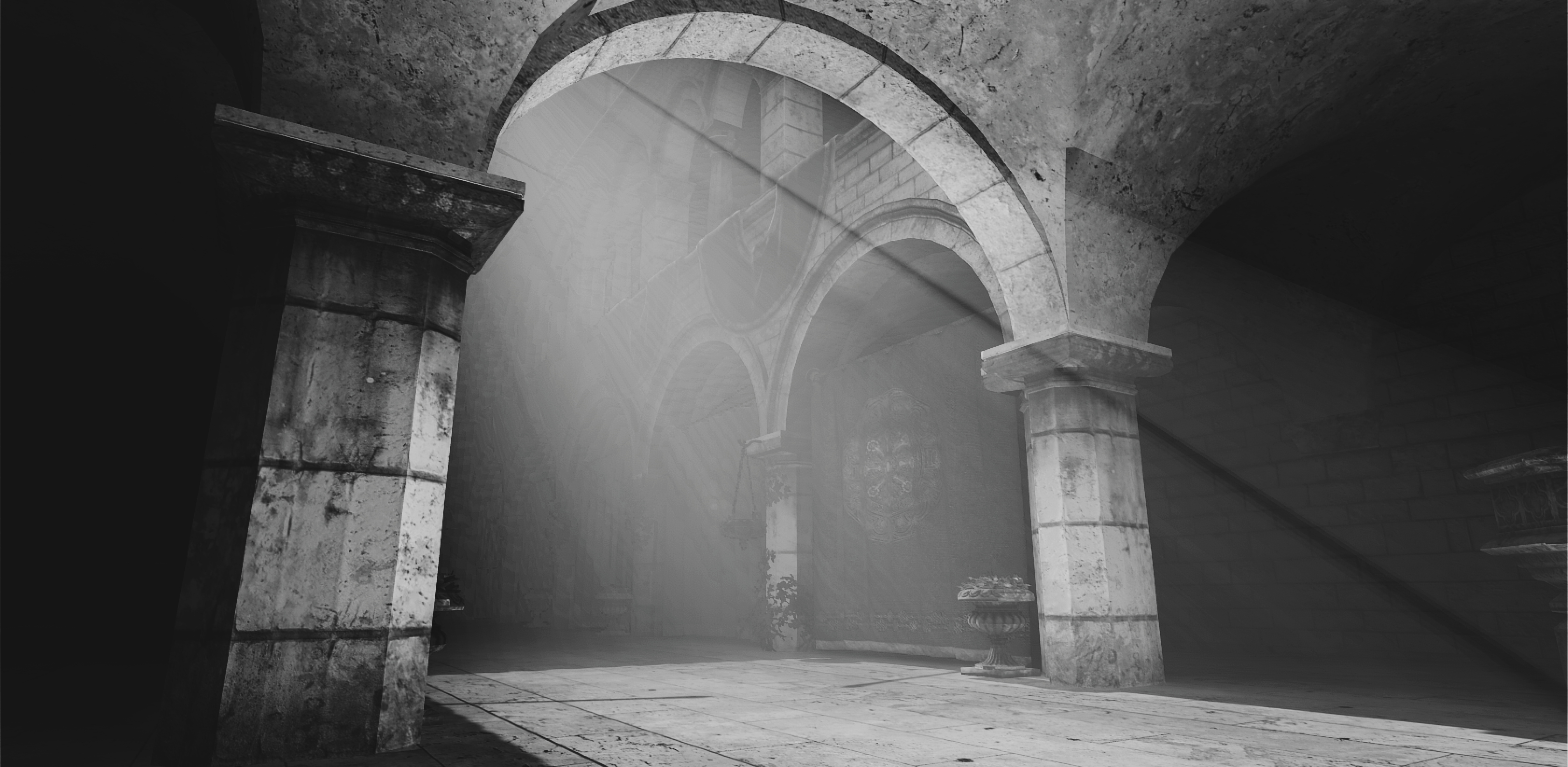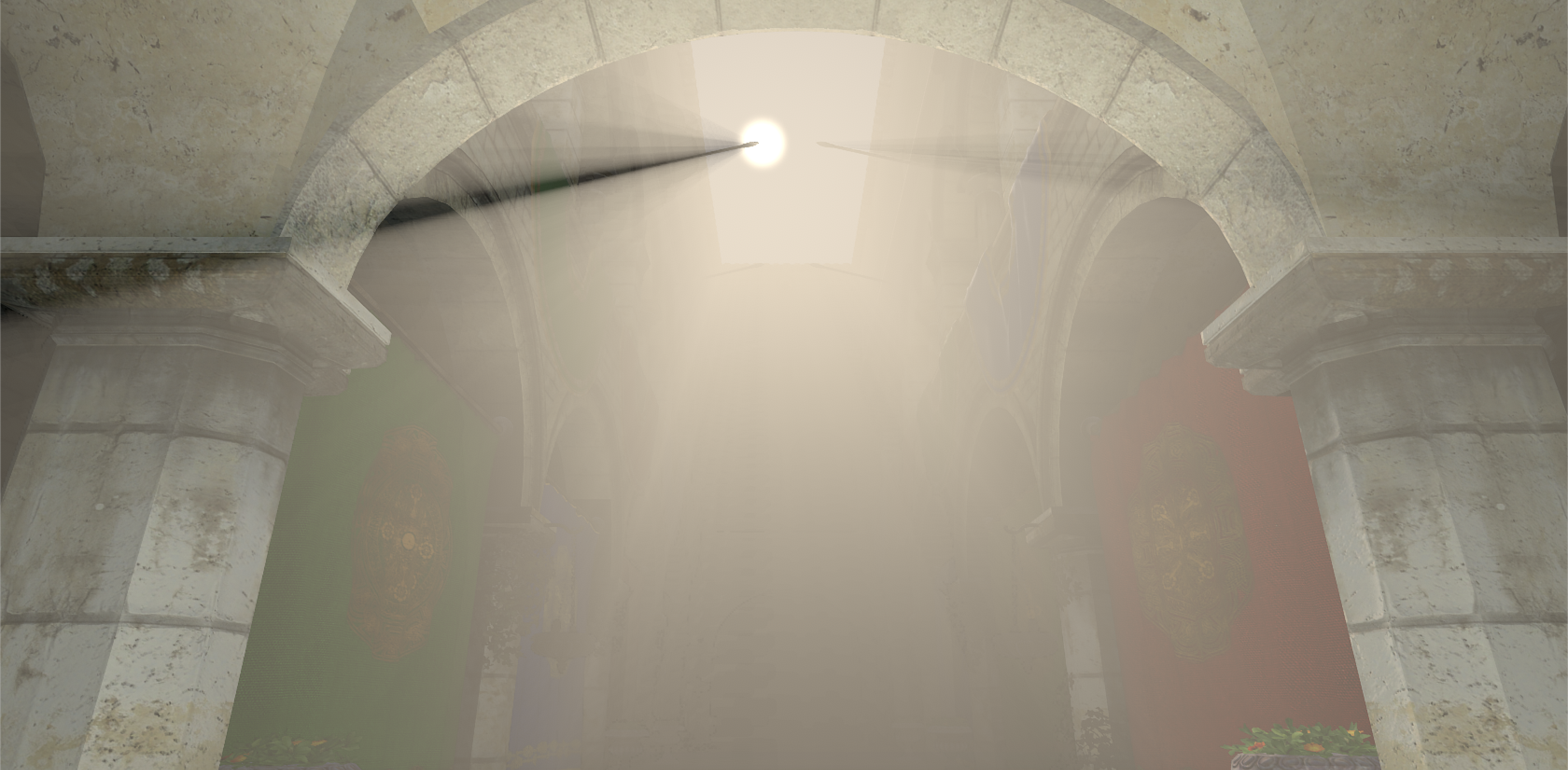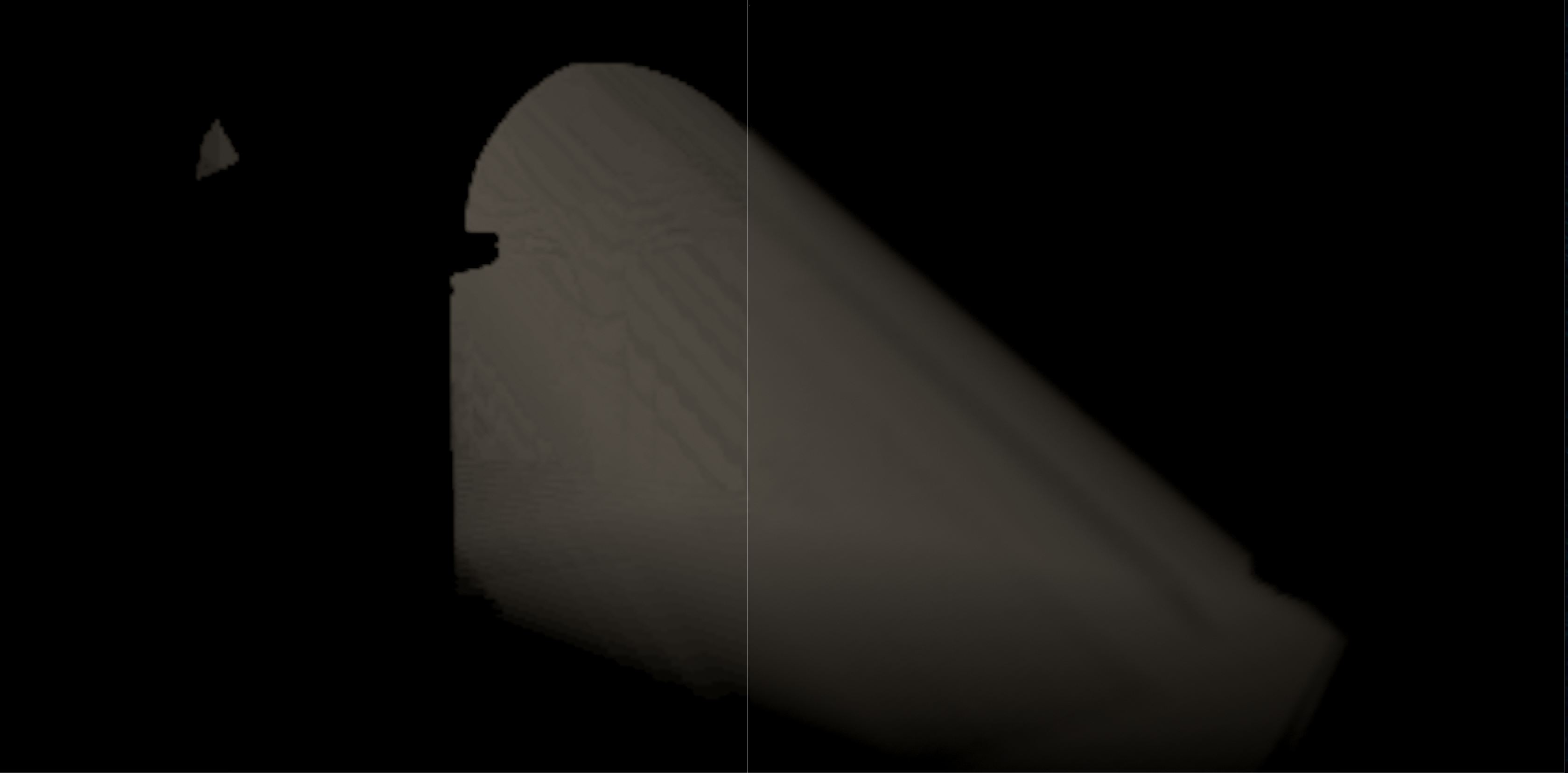# Volumetric Lighting

A project for Dr. Stephen Guy’s class Game Engine Technologies.

Our directive was to expand upon a topic we found interesting from earlier in the course. I chose to explore real-time volumetric lighting.

Below I give a brief explanation of the math that governs how light behaves in a participating medium. I also discuss my own implementation in a game engine, and provide platform-agnostic pseudo-code.

## MediaA black and white image of my implementation.

A directional light moving through the Sponza atrium.

A point light moving through the Sponza atrium at night.

A spot light moving through the Sponza atrium at night.

## 1. Light Propagation in Participating Media

Before light reaches your eye, it interacts with particles suspended in the air. Because the particles in the air alter the trajectory of the light that passes through them, we call the air a “participating medium”.

Depending on the composition of the medium (and the concentration of the particles within it), any of the following events could occur and alter the light before it reaches your eye.

• Absorption: light could be absorbed by a particle and converted into a different form of energy such as heat.
• Out-scattering: light could hit a particle and bounce in a different direction away from your eye.
• In-scattering: light from a different direction could hit a particle and bounce towards your eye.

### 1.1 Light Propagation in Participating MediaLight interacts with the air before it reaches your eye.

The light reaching your eye at point $p_a$ after traveling through a medium from point $p_b$ is described by the following equation:

$L = L_{reduced} + L_{inscattered}$

Where $L_{reduced}$ is the light lost between $p_a$ and $p_b$ due to absorption and out-scattering, and $L_{inscattered}$ is the light gained between $p_a$ and $p_b$ due to in-scattering.

### 1.2 Reduced RadianceThe above image, with only the reduced radiance visible. Notice that the further back the scene goes, the darker the image gets. As the distance increases, so too does the probability that light will be absorbed or out-scattered.

As light travels to your eye in a participating medium, a portion of it will be lost due to absorption and out-scattering. We call the light that reaches your eye the “reduced radiance”. The reduced radiance is defined as:

$L_{reduced} = I(p_b, -v) \times T(p_a, p_b)$

Where $I$ Is the original light at point $p_b$ traveling towards your eye (opposite the view ray) in direction $-v = -(p_b - p_a)$, and $T$ is the transmittance (a measure of the original light that reaches your eye as a function of the distance travelled). The transmittance between two points is defined as:

$T(p_a, p_b) = e^{-\int_{p_a}^{p_b}\sigma_e(p)dp}$

Where $\sigma_{e} = \sigma_{a} + \sigma_{s}$ is the extinction coefficient, equal to the sum of the absorption coefficient $\sigma_{a}$ and scattering coefficient $\sigma_{s}$. The absorption and scattering coefficients are properties inherent to the medium, and roughly describe the probability of an absorbtion and/or scattering event occuring.

It is worth noting that the integral portion of the transmittance equation is sometimes refered to as the “optical depth”.

### 1.3 In-scatteringThe above image, with only the in-scattered light visible.

Light that might not have been travelling towards you in a medium may hit a particle and change its course towards your eye. The accumulation of these rogue light rays is responsible for the “god ray” effect. The in-scattered light is defined as:

$L_{inscattered} = \int_{p_a}^{p_b}T(p_a, p) \times \sigma_s(p) \times L_i(p, v) dp$

Where $L_i$ describes the light at point $p$ scattered into the view ray $v = p_{b} - p_{a}$. It is defined as:

$L_i(p, v) = \int_{\Omega} I(p, \omega) \times F(v, \omega) d\omega$

And where $\Omega$ represents the sphere of all possible directions, $I$ is the light at $p$ traveling from direction $\omega$, and $F$ is the phase function. The phase function is inherent to the medium, and describes the probability of light from a given direction scattering into the viewing ray $v$.

## 2. Implementation Details

I integrated my implementation in the Unity engine.

### 2.1 My Approach

My initial idea was to render a full-screen quad, and perform the volumetric lighting calculations in screen-space. For each fragment, I sampled the depth buffer to reconstruct the world-space position. I then traced a ray from the camera to that position, and integrated the above equations along the ray.

That worked well, and was relatively easy to implement. Unfortunately, I quickly discovered some major limitations. To my current knowledge, Unity only provides lighting information for point and spot lights during certain passes in the rendering pipeline. By the time my screen-space shader ran, I was unable to access any meaningful point or spot light data.

Additionally, tracing rays proved to be more expensive than I initially thought. Even at a quarter resolution, the performance was still sluggish. I was forced to reduce the amount of samples along the ray from 128 (which provided almost perfect visual quality) to 32. This introduced unacceptable visual artifacts in the form of banding.

To alleviate the banding, I offset the start position of each ray by a different random, tiny amount each frame. The banding was eliminated, at the expense of noise. This was still a preferable trade-off, since Unity’s temporal anti-aliasing system was able to smooth out the noise rather welll.Banding artifacts due to insufficiently low samples (left). Reduced banding artifacts by offsetting each ray by a random amount (right).

Unfortunately, I still wasn’t able to access the point and spot light data so I turned to a different approach.

I re-wrote my screen-space shader as a regular shader and applied it to a cube mesh. Because it was attached to a mesh, the shader executed with rest of the meshes and the lighting information was correctly set. I also enabled front-face culling, so that the viewer could still appear to go inside the “volume” mesh. Because this approach was not done in screen-space I was unable to perform the volumetric lighting calculations at a downsampled resolution so the performance was much worse than my initial attempt.

Integrating my implementation in Unity was an interesting experience. At times I definitely felt as though I was fighting the engine. It made me curious to write my own engine. That way I could have full control over what lighting information was generated, and when.

### 2.2 Pseudocode

I will attempt to keep the pseudocode below implementation agnostic, although I will assume that you have certain buffers available to you (depth, color, and world position).

The first step is calculating the ray origin ($p_a$), direction ($v$), and end ($p_b$). The origin is the world-space camera position. The end is the world-space fragment position. (This could be supplied in a buffer as part of a deferred rendering pipeline, or recalculating by multiplying the value in the depth buffer by the inverse view-projection matrix).

// Calculate the fragment position from the world position buffer,
// and get the camera position.
vec3 fragPos = SAMPLE_WORLD_POSITION_BUFFER(screenCoord);
vec3 viewPos = GetWorldSpaceCameraPos();

// Calculate the viewing direction (unit vector) and
// distance to the fragment from the camera.
vec3 viewDir = fragPos - viewPos;
float fragDist = length(viewDir);
viewDir /= fragDist;


The next step is declaring accumulator values for the optical depth and in-scattered light. We also need to decide how many times to step through the volume.

// Accumulators.
float opticalDepth = 0.0;
vec3 inscatteredRadiance = vec3(0.0, 0.0, 0.0);

// Higher step count yields better results,
// but is more expensive.
const int STEPS = 32;
const float DP = fragDist / (float)STEPS;


Then we must actually step through the volume and perform the integrations.

for (int i = 0; i < STEPS; i++)
{
// How far along the ray we are, as a 0...1 percentage.
float percent = i / (float)(STEPS-1.0);

// The current position along the ray.
vec3 p = lerp(viewPos, fragPos, percent);
}


Within the loop, we also need to get the coefficients at the current position in the volume. These could be supplied as an analytical function (e.g. height fog), by sampling a 3D noise texture, or by some other method. We also need to calculate the transmittance at the current position along the ray.

// Sample the coefficients at the current position.
float absorption = GetAbsorptionCoefficientAt(p);
float scattering = GetScatteringCoefficientAt(p);
float extinction = absorption + scattering;

// Calculate the transmittance up to this point in the ray.
float curTransmittance = exp(-opticalDepth);


We also need to calculate the Li term, all of the light at the current position scattered into the viewing ray. In the real world light would be coming into the point from every direction and we would have to integrate all of it. In this implementation, we only have light coming in from a discrete set of lights, so we can just loop over those.

// Calculate the in-scattered light at the current position.
vec3 Li = vec3(0.0, 0.0, 0.0);
for (int l = 0; l < LIGHTS.length; l++)
{
// GetLightVisibility() just returns whether the point p is
// in shadow or not, from the perspective of the light l. This
// is typically done by sampling the light's shadow map.
vec3 curLi = GetLightVisibility(p, l) * LIGHTS[l].color;

// The phase function relies on the angular difference
// between the view direction and the light direction.
// The Henyey-Greenstein phase function is commonly used
// because it can approximate many different types of
// media with a single parameter G = [-1, 1].
float theta = acos(dot(viewDir, LIGHTS[l].dir));
curLi *= HenyeyGreenstein(theta, G);

// Accumulate Li.
Li += curLi;
}


Finally, we need to actually integrate the accumulators along the ray.

// Integrate along the ray.
opticalDepth += extinction * DP;
inscatteredRadiance += curTransmittance * scattering * Li * DP;

// End of integration for-loop.


After the loop is done, we need to calculate the final transmittance and return the final radiance.

// Calculate the final transmittance.
float transmittance = exp(-opticalDepth);

// Sample the color buffer and calculate the reduced radiance.
vec3 fragRadiance = SAMPLE_COLOR_BUFFER(screenCoord);
vec3 reducedRadiance = fragRadiance * transmittance;

// Return the reduced + inscattered radiance.
return vec4(reducedRadiance + inscatteredRadiance, 1.0);


## 3. Source Code / Credit

• The source code is available to download here.
• I borrowed a ray-AABB intersection algorithm from here.
• I used a set of free blue noise textures by Christoph Peters.
• The 3D model of the Sponza Atrium is from Morgan McGuire’s Computer Graphics Archive.
• Finally, Wojciech Jarosz’s dissertation on light transport in participating media was an invaluable resource.